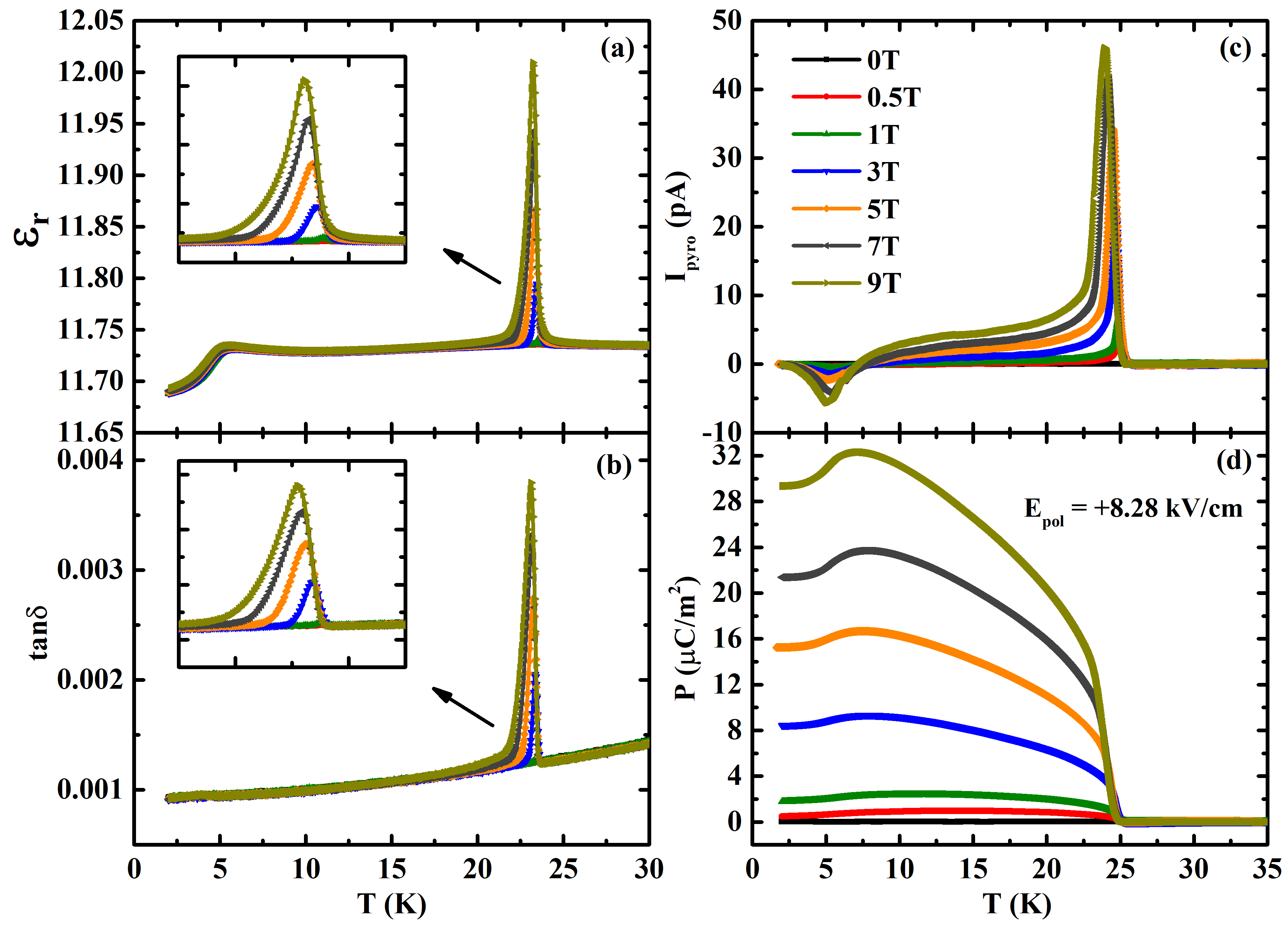# Linear magnetoelectric effect in antiferromagnetic Sm2BaCuO5

#### Linear magnetoelectric effect in antiferromagnetic Sm2BaCuO5

We report the discovery of linear magnetoelectric effect in the well-known green-phase compound, Sm2BaCuO5, which crystallizes in the centrosymmetric orthorhombic (Pnma) structure. Magnetization and specific heat measurements reveal the long-range antiferromagnetic ordering of Cu2+ and Sm3+ ions moments at TN1=23K and TN2=5K, respectively. Applied magnetic field induces dielectric anomaly at TN1 whose magnitude increases with field, which results in significant (1.7%) magnetocapacitance effect. On the other hand, the dielectric anomaly observed in zero-applied magnetic field at TN2 shows a small (0.4%) magnetocapacitance effect. Interestingly, applied magnetic field induces an electric polarizaiton, below TN1, that varies linearly up to the maximum applied field of 9 T with the magnetoelectric coefficient α ∼ 4.4 ps/m, demonstrating high magnetoelectric coupling. Below TN2, the electric polarization decreases from 35 to 29 μC/m2 at 2 K and 9 T due to ordering of Sm sublattice. The observed linear magnetoelectricity in Sm2BaCuO5 is explained using symmetry analysis.Figure (a), (b) Temperature-dependent dielectric constant and loss factor measured under different magnetic fields with 50-kHz frequency. (c), (d) Leakage current subtracted pyroelectric current as a function of temperature under different magnetic fields and poling electric field E = +8.28 kV/cm and corresponding polarization obtained by integrating pyrocurrent with respect to time.